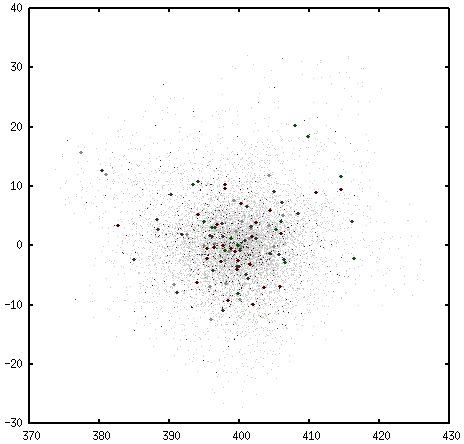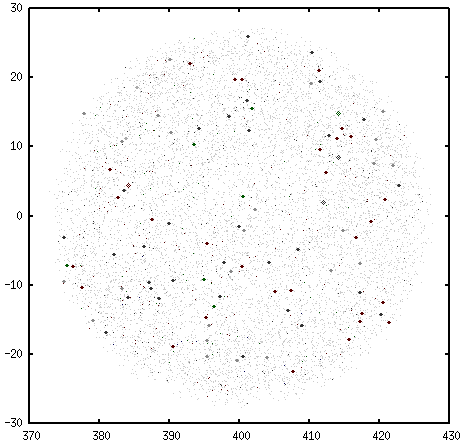# Daniel P. Barron

### The Circle Explore Method.

Wednesday, February 8, 2017

I had previously described how to make your Eulora character explore like a drunk. This is a very useful method when the area you are trying to target is closer to a point. The drunk explorer has a strong preference for gathering closer to the starting location; even at large `size` settings, the distribution will still put most of the attempts near the center. I have created a graph of 10`000 explore attempts using the drunk method in order to demonstrate this property. It looks like a fuzzy paintbrush tool from an image manipulation program.

Figure 1At `size` value `25`, this method remarkably covers an area similar to that of a circle bearing a radius of the same number. You will notice that most of the points are located within a circle with radius 10, although a few outliers i cause the graph to stretch vertically. I find this useful for gathering higher-end uncommon ii resources like wooly mushroom, which are usually found in areas of about this size. Here is a graph, same number of attempts, using the `circle` explore method.

Figure 2Those fuzzy diamonds are ordinary claims. Figure 1 is a sample of attempts over the same area, yet it did not find any ordinaries. Thus is demonstrated the usefulness of the circle explore method. If you don't know an area so well, except that it generally has some of the things you like, the `circle` method will give you an accurate survey. Since it's not biased towards any one point, the frequency of smalls and ordinaries in a given sub-region in this area relates to something inherent in the land itself. We can see from the survey in Figure 2 that it might be profitable to focus on coordinates `415, 10`, where three ordinaries were found.

It's just like the drunk method, but with a different set of rules concerning how the character turns after each attempt. Edit `Eulora/src/client/foxybot/botactivity.cpp` and add the following to the switch contained within `MovementStrategy::Move`.

```        case EXPLORE_CIRCLE:
{
if ((double)(pow(currPos.x - startPos.x, 2) + pow(currPos.z - startPos.z, 2)) > (double)pow(size, 2))
{
worldHandler::TurnToPoint(startPos.x, startPos.z);
worldHandler::Turn((3 * M_PI) / 2);
worldHandler::Turn(((double)rand() / (double)RAND_MAX) * M_PI);
}

worldHandler::StepForward();

break;
}
```

You may also need to add this bit to the start of the same function.

```void MovementStrategy::Move(int movesDone)
{
currPos = worldHandler::GetCurrentPos();

if (movesDone < 1) startPos = currPos;
```

As you continue to make changes to your bot, it'll come in handy that these variables get set outside of the scope of any one particular explore method. There's one more thing you need to add to this file, and that's the part where the method gets passed into the bot by the user.

```                if (words.GetCount() > 0)
{
csString s;
words.GetString(0, s);
if (s.CompareNoCase(csString("line")))
strat = EXPLORE_LINE;
else if (s.CompareNoCase(csString("grid")))
strat = EXPLORE_GRID;
else if (s.CompareNoCase(csString("drunk")))
strat = EXPLORE_DRUNK;
else if (s.CompareNoCase(csString("circle")))
strat = EXPLORE_CIRCLE;
else if (s.CompareNoCase(csString("snake")))
strat = EXPLORE_SNAKE;
else if (s.CompareNoCase(csString("point")))
strat = EXPLORE_DRUNK;
else if (s.CompareNoCase(csString("test")))
strat = EXPLORE_TEST;
else
{
strat = EXPLORE_LINE;
OutputMsg(csString("UNKNOWN MOVEMENT STRATEGY specified, so will use default (line)."));
}
}
```

As you can see, I've got a few others in there; I'll leave the design of these other methods as an exercise for you. iii One more thing you need to change before recompilation. Go into `Eulora/src/client/foxybot/botactivity.h` and add a line to the `EXPLORE_STRATEGIES` enum.

```enum EXPLORE_STRATEGIES
{
EXPLORE_GRID = 2,
EXPLORE_LINE = 3,
EXPLORE_DRUNK = 4,
EXPLORE_CIRCLE = 5,
EXPLORE_SNAKE = 6,
EXPLORE_POINT = 7,
EXPLORE_TEST = 9
};
```

Once you've got those files saved, run `jam -aq client` in `~/development/Eulora/` and restart your game.

1. This is part of the fun with drunken exploring. Sometimes your character will get quite far from the origin. When this happens it's not unlike witnessing the roulette wheel come up red ten times in a row. ^
2. Here is the way I sort harvestable resources into categories of rarity: if it is always profitable to build as an ordinary claim, it is rare; if it is always profitable to build as a small claim, it is uncommon; everything else is common. ^
3. Mostly because I consider them to be experimental; they are not nearly as reliable as `drunk` and `circle` have proven to be. But you took the time to click down to the footnote, so here's a quick description. With `snake` it's supposed to go all over the place, snaking to and fro based on the slope of its movement -- that is, whether the character has moved up or down on the Y-axis since the last try.

```        case EXPLORE_SNAKE:
{
if (slope > 0)
{
slope = lastPos.y - currPos.y;

if (slope > 0)
worldHandler::Turn(((double)rand() / (double)RAND_MAX) * (M_PI/size));
else
worldHandler::Turn((M_PI * 2) - ((double)rand() / (double)RAND_MAX) * (M_PI / size));
}
else
{
slope = lastPos.y - currPos.y;

if (slope > 0)
worldHandler::Turn((M_PI * 2) - ((double)rand() / (double)RAND_MAX) * (M_PI / size));
else
worldHandler::Turn(((double)rand() / (double)RAND_MAX) * (M_PI / size));
}

worldHandler::StepForward();

break;
}
```